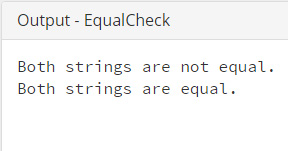# Java Program to Compare Two Strings

This Java program is used to demonstrates a comparison of two strings.

• Java equals() method is used to compare strings.
• Java equalsIgnoreCase() method can ignore the case.
• We cannot use == operator to compare two strings.
Example:
``````public class EqualCheck {
public static void main(String args[]){
String a = "AVATAR";
String b = "avatar";

if(a.equals(b)){
System.out.println("Both strings are equal.");
} else {
System.out.println("Both strings are not equal.");
}

if(a.equalsIgnoreCase(b)){
System.out.println("Both strings are equal.");
} else {
System.out.println("Both strings are not equal.");
}
}
}
``````
Program Output:Here's a detailed explanation of what's happening inside this code snippet:

Explanation:
First of all, a class named EqualCheck is declared with the keyword public. Public designates that the class can be accessed from anywhere within the program.

Within this class, the main() method is defined. The main() method has two String variables. These are:

• String a = "AVATAR";
• String b = "avatar";

First String type variable a is storing the string value AVATAR, and the second variable b is storing the string value avatar.

It is to be noted that both variables a and b will generate different ASCII (American Standard Code for Information Interchange) values, and the string comparison is checked based on the ASCII values among two or more strings.

a.equals(b) is a pre-defined method of Java String Class which checks whether two given and initialized strings are equal or not. If found equal, the statement System.out.println ("Both strings are equal."); will get printed else this statement System.out.println ("Both strings are not equal."); gets printed.

Again, it is to be noted that the comparison is case-sensitive. So to perform a comparison which ignores case differences, you have to use equalsIgnoreCase() method. As it compares two strings, it considers A-Z to be the same as a-z.

In this Java program, it ignores the upper and lower case issue and compares both the strings.